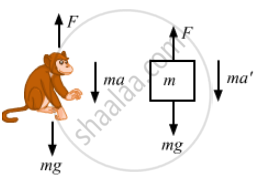# A Monkey is Climbing on a Rope that Goes Over a Smooth Light Pulley and Supports a Block of Equal Mass at the Other End in the Followin - Physics

Sum

A monkey is climbing on a rope that goes over a smooth light pulley and supports a block of equal mass at the other end in the following figure. Show that whatever force the monkey exerts on the rope, the monkey and the block move in the same direction with equal acceleration. If initially both were at rest, their separation will not change as time passes.#### Solution

Suppose the monkey accelerates upward with acceleration a and the block accelerates downward with acceleration a'.
Let force exerted by the monkey be F.From the free-body diagram of the monkey, we get:
F− mg − ma = 0    ...(i)
⇒ F = mg + ma
Again, from the free-body diagram of the block,
F + ma' − mg = 0
mg + ma + ma' − mg = 0            [From (i)]
⇒ ma = −ma'
⇒ a = −a'
If acceleration −a'  is in downward direction then the acceleration a' will be in upward direction.
This implies that the block and the monkey move in the same direction with equal acceleration.
If initially they were at rest (no force is exerted by the monkey), then their separation will not change as time passes because both are moving  same direction with equal acceleration.

Concept: Newton’s Second Law of Motion
Is there an error in this question or solution?

#### APPEARS IN

HC Verma Class 11, Class 12 Concepts of Physics Vol. 1
Chapter 5 Newton's Laws of Motion
Q 37 | Page 82